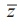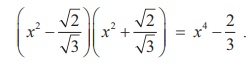Home | | Maths 12th Std | Nature of Roots and Nature of Coefficients of Polynomial Equations

# Nature of Roots and Nature of Coefficients of Polynomial Equations

Nature of Roots and Nature of Coefficients of Polynomial Equations

Nature of Roots and Nature of Coefficients of Polynomial Equations

## 1. Imaginary Roots

For a quadratic equation with real coefficients, if α + iβ is a root, then α iβ is also a root. In this section we shall prove that this is true for higher degree polynomials as well.

We now prove one of the very important theorems in the theory of equations.

## Theorem 3.2 (Complex Conjugate Root Theorem)

If a complex number z0  is a root of a polynomial equation with real coefficients, then its complex conjugate0 is also a root.

Proof

Let P ( x) = an xn + an-1 xn-1 +...+ a1 x + ao = be a polynomial equation with real coefficients.

Let z0 be a root of this polynomial equation. So, P( z0) = 0. NowThat is P(0 ) = 0 ; this implies that whenever z0 is a root (i.e. P( z0 )=0), its conjugate0  is also a root.

If one asks whether 2 is a complex number, many students hesitate to say “yes”. As every integer is a rational number, we know that every real number is also a complex number. So to clearly specify a complex number that is not a real number, that is to specify numbers of form α + iβ  with  β ± 0 , we use the term “non-real complex number”. Some authors call such a number an imaginary number.

Remark 1

Let z0  = α + with β ± 0 . Then0   = α - . If α + is a root of a polynomial equation P(x) = 0 with real coefficients, then by Complex Conjugate Root Theorem,  α - iβ   is also a root of P(x) = 0 .

Usually the above statement will be stated as complex roots occur in pairs; but actually it means that non-real complex roots or imaginary roots occur as conjugate pairs, being the coefficients of the polynomial equation are real.

Remark 2

From this we see that any odd degree polynomial equation with real coefficients has at least one real root; in fact, the number of real roots of an odd degree polynomial equation with real coefficients is always an odd number. Similarly the number of real roots of an even degree polynomial equation with real coefficients is always an even number.

Example 3.8

Find the monic polynomial equation of minimum degree with real coefficients having 2 - √3 i as a root.

### Solution

Since 2 - √3i is a root of the required polynomial equation with real coefficients, 2 + √3i is also a root. Hence the sum of the roots is 4 and the product of the roots is 7 . Thus x2 - 4x + 7 = 0 is the required monic polynomial equation.

## 2. Irrational Roots

If we further restrict the coefficients of the quadratic equation ax2 + bx + c = 0 to be rational, we get some interesting results. Let us consider a quadratic equation ax2 + bx + c = 0 with a , b, and c rational. As usual let Δ = b2 4ac and let r1 and r2 be the roots. In this case, when Δ = 0 , we have r1 = r2 ; this root is not only real, it is in fact a rational number.

When Δ is positive, then no doubt that √Δ exists in R and we get two distinct real roots. But √Δ will be a rational number for certain values of a, b, and c , and it is an irrational number for other values of a, b , and c .

If √Δ is rational, then both r1 and r2 are rational.

If √Δ is irrational, then both r1 and r2 are irrational.

Immediately we have a question. If Δ > 0 , when will √Δ be rational and when will it be irrational? To answer this question, first we observe that Δ is rational, as the coefficients are rational numbers. So Δ = m/n for some positive integers m and n with (m, n) = 1 where (m, n) denotes the greatest common divisor of m and n. It is now easy to understand that √Δ is rational if and only if both m and n are perfect squares. Also, √Δ is irrational if and only if at least one of m and n is not a perfect square.

We are familiar with irrational numbers of the type p + √q where p and q are rational numbers and √q is irrational. Such numbers are called surds. As in the case of imaginary roots, we can prove that if p + √q  is a root of a polynomial, then p - √q  is also a root of the same polynomial equation, when all the coefficients are rational numbers. Though this is true for polynomial equation of any degree and can be proved using the technique used in the proof of imaginary roots, we state and prove this only for a quadratic equation in Theorem 3.3.

Before proving the theorem, we recall that if a and b are rational numbers and c is an irrational number such that a + bc is a rational number, then b must be 0 ; further if a + bc = 0 , then a and b must be 0.

For instance, if a + b√2 Q, then b must be 0 , and if a + b√2 = 0 then a = b = 0 . Now we state and prove a general result as given below.

### Theorem 3.3

Let  p  and q be rational numbers such that √q  is irrational. If p +q is a root of a quadratic equation with rational coefficients, then p q is also a root of the same equation.

Proof

We prove the theorem by assuming that the quadratic equation is a monic polynomial equation.

The result for non-monic polynomial equation can be proved in a similar way.

Let p and q be rational numbers such that √q is irrational. Let p + √q be a root of the equation x2 + bx + c = 0 where b and c are rational numbers.

Let α be the other root. Computing the sum of the roots, we get

α + p + √q = -b

and hence α + √q = -b - p Q. Taking -b - p as s , we have α + √q = s .

This implies that

α = s - √q.

Computing the product of the roots, gives

(s -√q )( p + √q) ) = c

and hence (sp - q) + (s - p) = c Q. Thus s - p = 0 . This implies that s = p and hence we get α = p – √q. So, the other root is p - √q.

### Remark

The statement of Theorem 3.3 may seem to be a little bit complicated. We should not be in a hurry to make the theorem short by writing “for a polynomial equation with rational coefficients, irrational roots occur in pairs. This is not true.

For instance, the equation x3 - 2 = 0 has only one irrational root, namely. 3√2. Of course, the other two roots are imaginary numbers (What are they?).

### Example 3.9

Find a polynomial equation of minimum degree with rational coefficients, having 2 - √3 as a root.

### Solution

Since 2 - √3 is a root and the coefficients are rational numbers, 2 + is also a root. A required polynomial equation is given by

2 - (Sum of the roots) x + Product of the roots = 0

and hence

x2 - 4x +1 = 0

is a required equation.

### Note

We note that the term “rational coefficients” is very important; otherwise, x - (2 - √3) = 0 will be a polynomial equation which has 2 - √3 as a root but not 2 + √3. We state the following result without proof.

### Theorem 3.4

Let p and q be rational numbers so that √p and √q are irrational numbers; further let one  of √p and √q be not a rational multiple of the other. If √p + √q is a root of a polynomial equation with rational coefficients, then √p - √q , - √p + √q , and - √p - √q are also roots of the same polynomial equation.

### Example 3.10

Form a polynomial equation with integer coefficients withas a root.

### Solution

Sinceis a root, x –is a factor. To remove the outermost square root, we take x +as another factor and find their productStill we didn’t achieve our goal. So we include another factor x2 + [√2/√3] and get the productSo, 3x4 - 2 = 0 is a required polynomial equation with the integer coefficients.

Now we identify the nature of roots of the given equation without solving the equation. The idea comes from the negativity, equality to 0, positivity of Δ = b2 - 4ac.

## 3. Rational Roots

If all the coefficients of a quadratic equation are integers, then Δ is an integer, and when it is positive, we have, √Δ is rational if, and only if,  Δ   is a perfect square. In other words, the equation   ax2 + bx + c = 0 with integer coefficients has rational roots, if, and only if, Δ is a perfect square.

What we discussed so far on polynomial equations of rational coefficients holds for polynomial equations with integer coefficients as well. In fact, multiplying the polynomial equation with rational coefficients, by a common multiple of the denominators of the coefficients, we get a polynomial equation of integer coefficients having the same roots. Of course, we have to handle this situation carefully. For instance, there is a monic polynomial equation of degree 1 with rational coefficients having 1/2 as a root, whereas there is no monic polynomial equation of any degree with integer coefficients having 1/2 as a root.

### Example 3.11

Show that the equation 2x2 - 6x + 7 = 0 cannot be satisfied by any real values of x.

### Solution

∆= b2 − 4ac = −20 < 0 . The roots are imaginary numbers.

Example 3.12

If x2 + 2 (k + 2)x + 9k = 0 has equal roots, find k.

Solution

Here Δ = b2  - 4ac = 0  for equal roots. This implies 4 (k + 2)2  = 4 (9) k .This implies k = 4 or 1.

Example 3.13

Show that, if p, q, r are rational, the roots of the equation x2 - 2 px + p2 - q2 + 2qr - r2 = 0 are rational.

Solution

The roots are rational if  Δ = b2  - 4ac = (-2 p)2  - 4 ( p2  - q2  + 2qr - r2 ) .

But this expression reduces to 4 (q2  - 2qr + r2 ) or 4 (q - r )2   which is a perfect square.  Hence the roots are rational.

Study Material, Lecturing Notes, Assignment, Reference, Wiki description explanation, brief detail
12th Mathematics : UNIT 3 : Theory of Equations : Nature of Roots and Nature of Coefficients of Polynomial Equations |